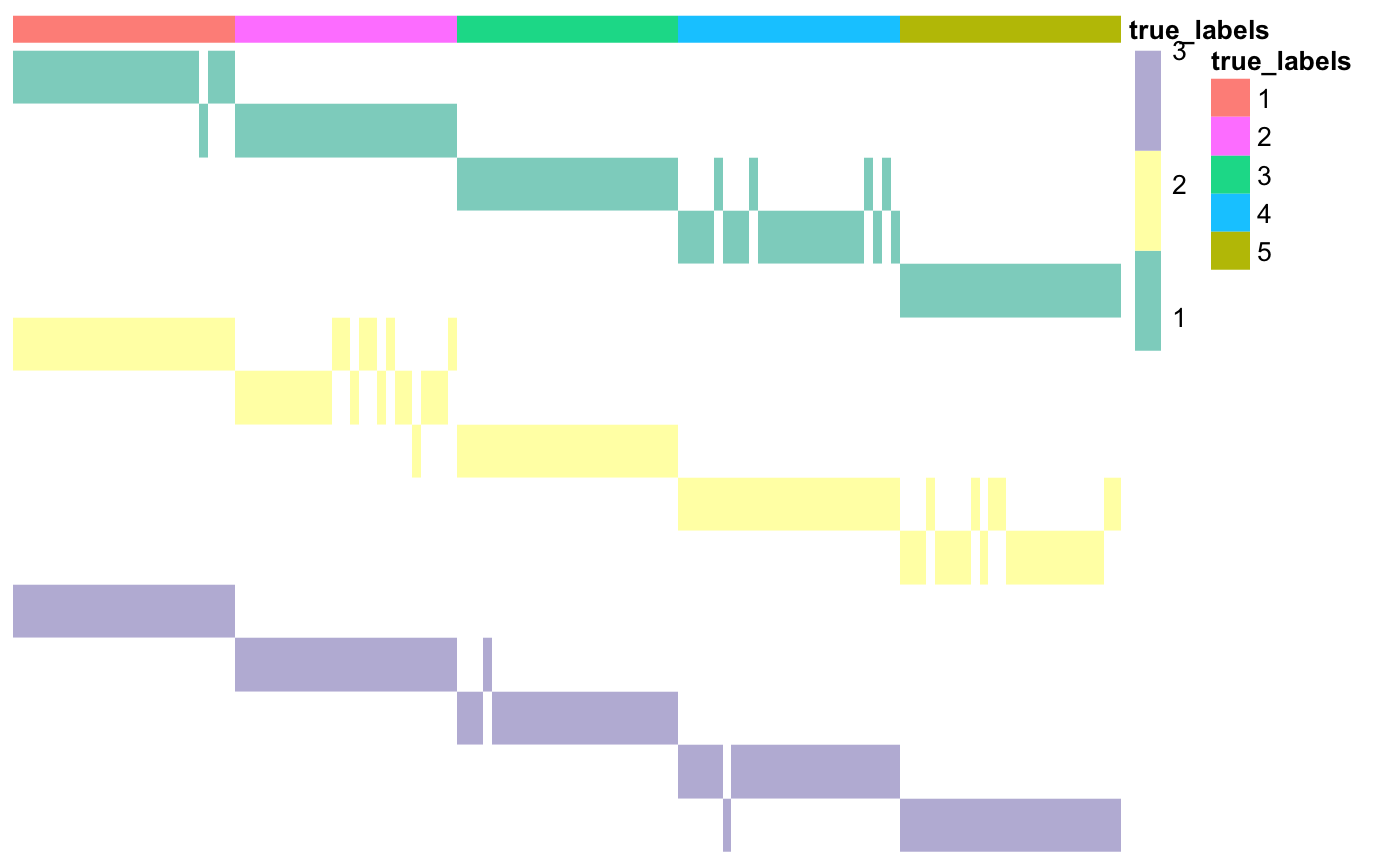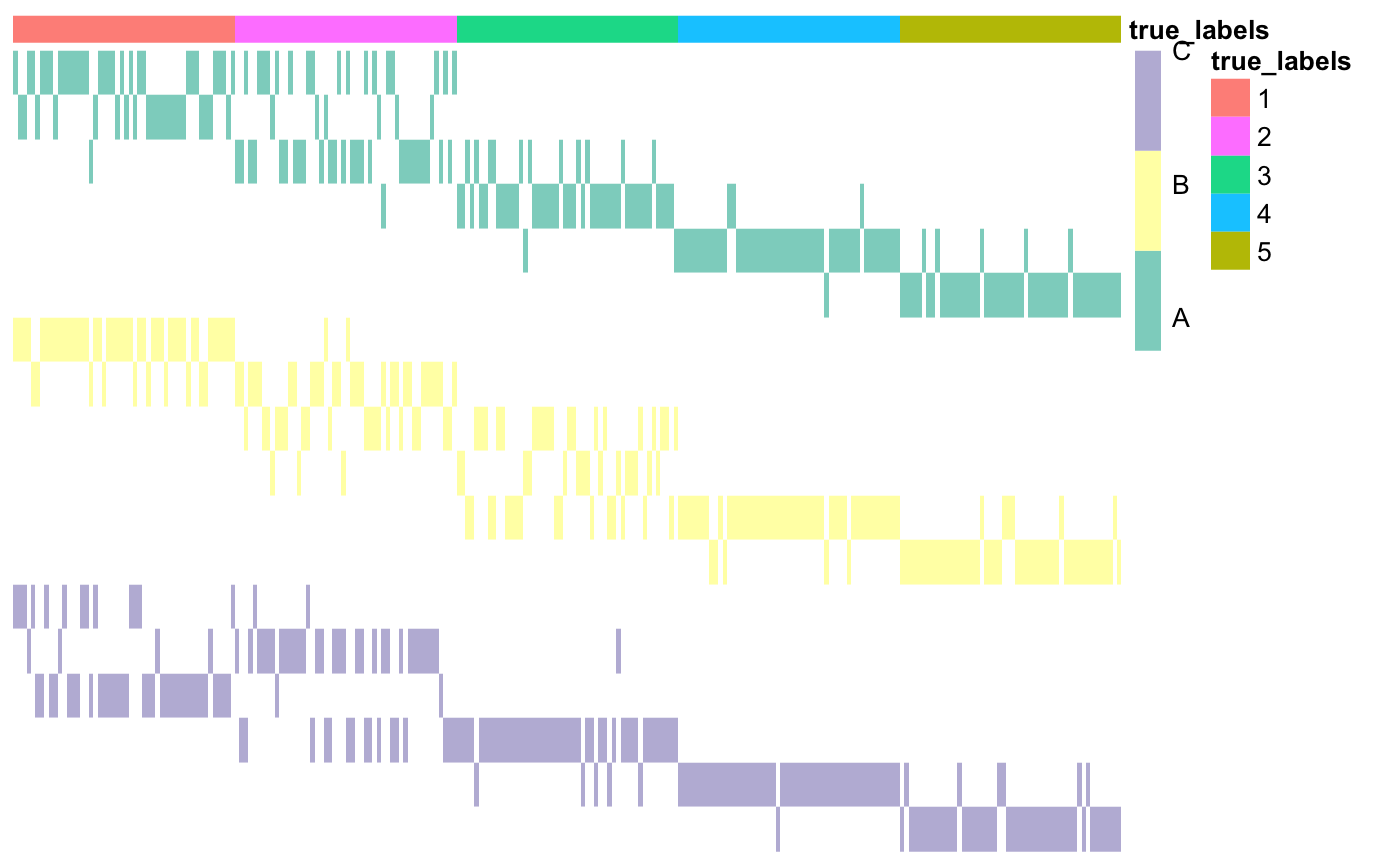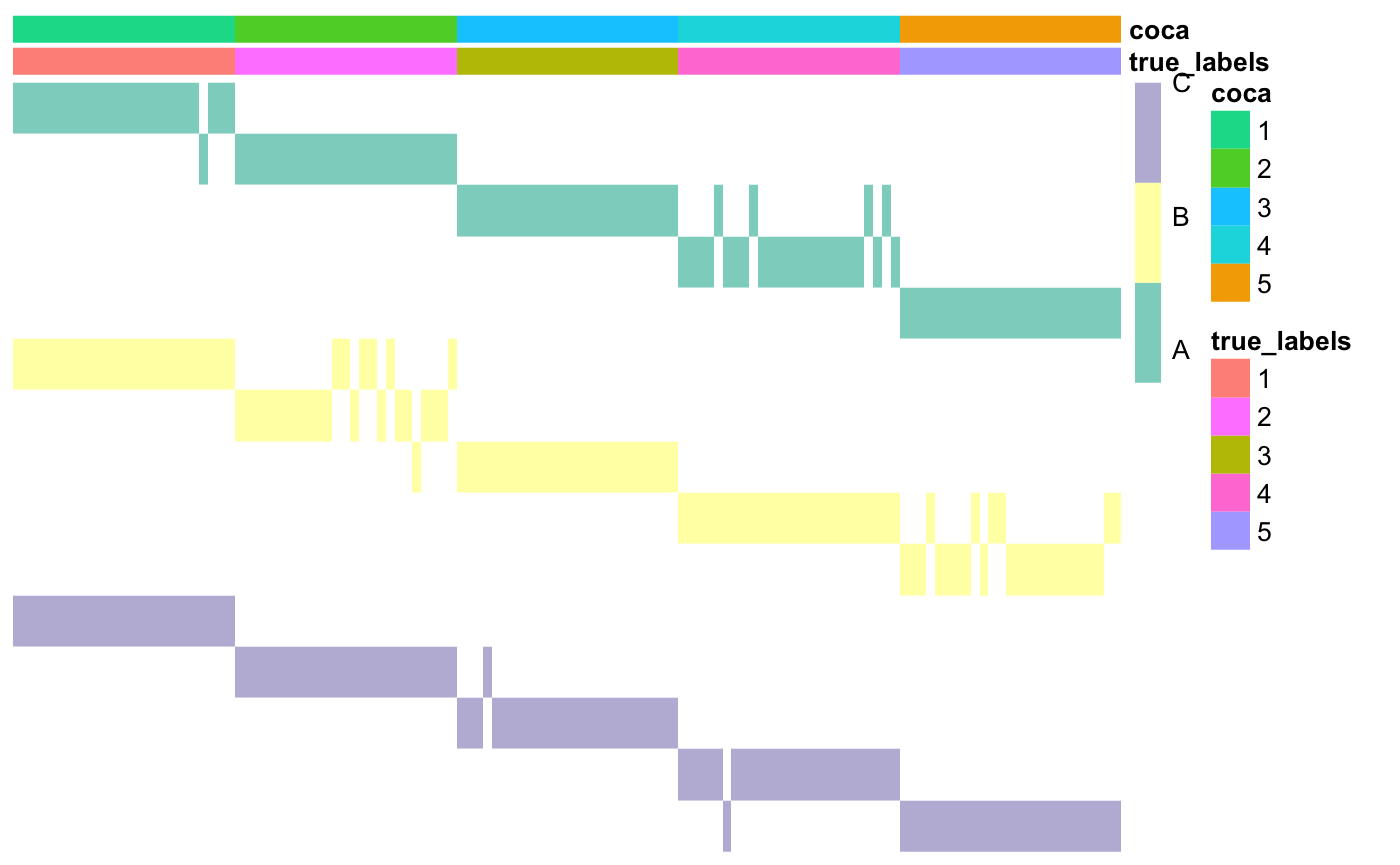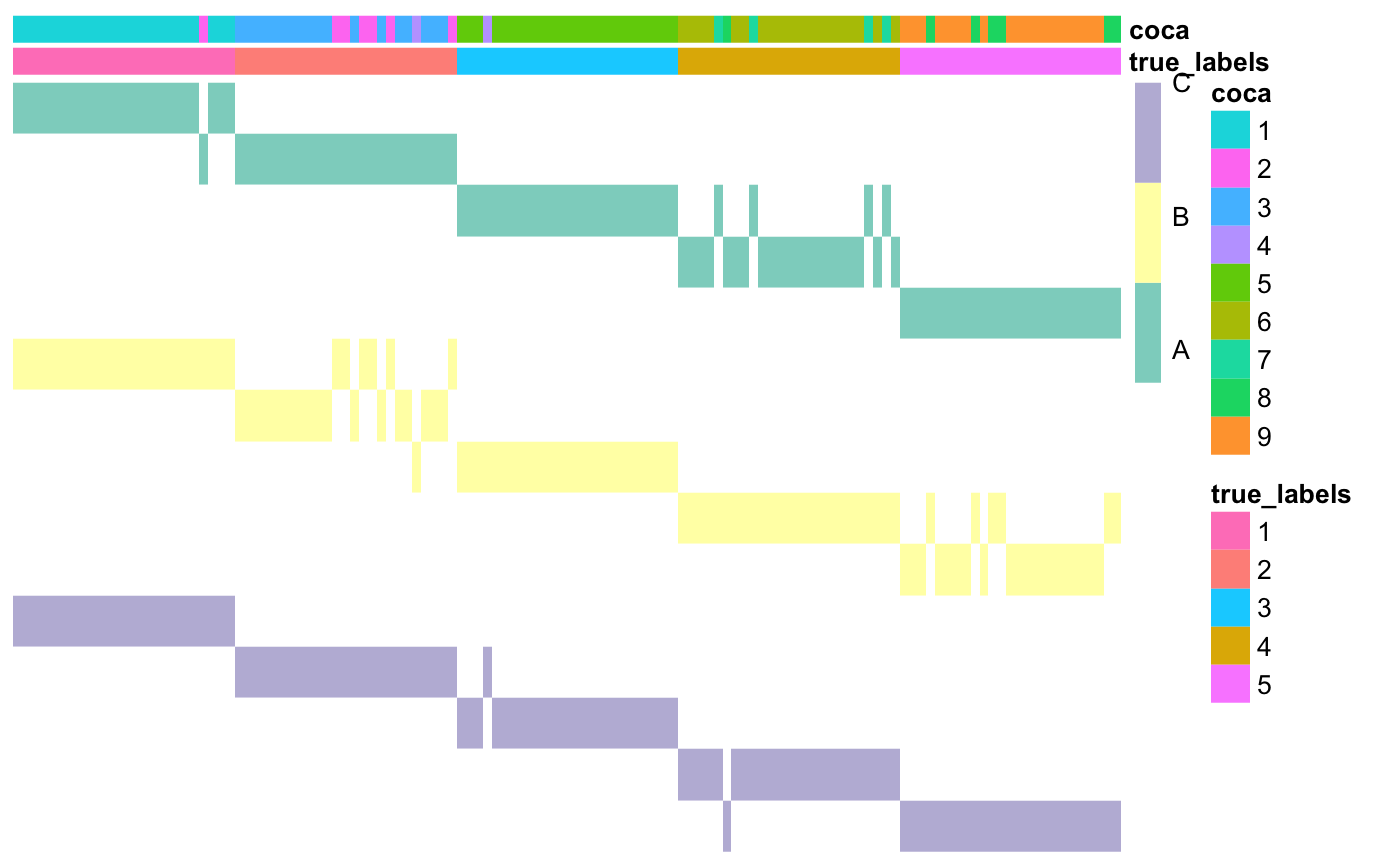# Introduction

The R package coca contains the functions needed to use COCA (Cluster-Of-Clusters Analysis), an integrative clustering method that was first introduced in a breast cancer study by The Cancer Genome Atlas in 2012 and quickly became a popular tool in cancer studies (see e.g. Hoadley et al. 2014 and Aure et al. 2017). It is based on (Monti et al., 2013), an algorithm that was initially developed to assess the stability of clusters obtained with any clustering algorithm.

The main goal of COCA is to summarise clusterings found in different ’omics datasets, by identifying a “global” clustering across the datasets that is intended to be in good agreement with the clustering structures identified in each of the individual datasets.

# Building the matrix of clusters

The first step of COCA is the construction of the Matrix-Of-Clusters (MOC). This is a binary matrix of size N x K, where K is the sum of the number of clusters Km in every dataset Xm. Therefore, to each column j of this matrix corresponds a cluster mk in dataset Xm. The (i,j)-th entry of the matrix of clusters is equal to one if data point i belongs to cluster mk in dataset Xm, and is equal to zero otherwise.For more details about how the matrix of clusters is built, please see Cabassi and Kirk (2018).

The function that can be used to build a matrix of clusters starting from a list of heterogeneous datasets (referring to the same observations) is buildMOC. In the example below, we assume to have three datasets with the same number of clusters, six. The clustering structure in each of those dataasets is found via k-means clustering.

### Load data
data <- list()
data[] <- as.matrix(read.csv(system.file("extdata",
"dataset1.csv", package = "coca"), row.names = 1))
data[] <- as.matrix(read.csv(system.file("extdata",
"dataset2.csv", package = "coca"), row.names = 1))
data[] <- as.matrix(read.csv(system.file("extdata",
"dataset3.csv", package = "coca"), row.names = 1))

### Build matrix of clusters
outputBuildMOC <- buildMOC(data, M = 3, K = 6, distances = "cor")

### Extract matrix of clusters and dataset indicator vector
moc <- outputBuildMOC$moc datasetIndicator <- outputBuildMOC$datasetIndicator

The package also contains a function that can be used to plot the resulting Matrix-Of-Clusters, plotMOC. Here we use as annotations the true cluster labels, but in real applications annotations can be any dataframe with one element for each row of the matrix of clusters (the row names must correspond!). Please be aware that each column of the dataframe that contains categorical variables must be defined with as.factor() if you want each category to have a different colour (otherwise they will be treaded as continuous variables and each category will have a different shade of the same colour).

### Prepare annotations
true_labels <- as.matrix(read.csv(system.file("extdata", "cluster_labels.csv",
package = "coca"), row.names = 1))
annotations <- data.frame(true_labels = as.factor(true_labels))

### Plot matrix of clusters
plotMOC(moc, datasetIndicator, annotations = annotations)Here the datasets don’t have names, so they have been assigned integer numbers. If available, you can specify cluster names; this will make the row names easier to interpret.

### Prepare annotations
true_labels <- as.matrix(read.csv(system.file("extdata", "cluster_labels.csv",
package = "coca"), row.names = 1))
annotations <- data.frame(true_labels = as.factor(true_labels))

### Set dataset names
datasetNames <- c(rep("A", 6), rep("B", 6), rep("C", 6))

### Plot matrix of clusters
plotMOC(moc, datasetIndicator, datasetNames = datasetNames,
annotations = annotations)As you can see, the first part of each row name corresponds to the dataset, the second one to the cluster index. Moreover, each colour in the main matrix corresponds to one dataset (here dataset A is green, dataset B is yellow, and so on).

# Cluster-Of-Clusters Analysis

The MOC matrix is then used as input to consensus clustering (CC), an algorithm that was developed by Monti et al. (2003) to assess cluster stability when analysing a single dataset. The resulting consensus matrix is then used as the similarity matrix for a hierarchical clustering method (or any other distance-based clustering algorithm). These last two steps of the COCA algorithm are contained in the coca() function.

### COCA

# Use COCA to find global clustering
coca <- coca(moc, K = 6, hclustMethod = "average")

# Compare clustering to the true labels
ari <- mclust::adjustedRandIndex(true_labels, coca$clusterLabels) ari ##  0.8163432 ### Plot the matrix of clusters with the newly found cluster labels annotations$coca <- as.factor(coca$clusterLabels) plotMOC(moc, datasetIndicator, datasetNames = datasetNames, annotations = annotations)Again, if the number of clusters is not know a priori, the user can delegate the choice of K to the coca() function. There are two methods available here: the silhouette, where the distance between data points i and j is defined as 1 minus the (i,j)-th kernel entry in the final kernel matrix, and the delta area under the curve, which is the method suggested by Monti et al. (2003). Please note that the properties of the latter have not been assessed yet. # Use COCA to find global clustering and chooose the number of clusters coca <- coca(moc, maxK = 10, hclustMethod = "average") # Compare clustering to the true labels ari <- mclust::adjustedRandIndex(true_labels, coca$clusterLabels)
ari
##  0.8163432
### Plot the matrix of clusters with the newly found cluster labels
annotations$coca <- as.factor(coca$clusterLabels)
plotMOC(moc, datasetIndicator, datasetNames = datasetNames,
annotations = annotations)